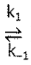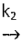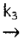# Problem: The proposed mechanism for a given reaction is as follows:Step One: 2 NO (g)    N2O2 (g)Step Two: H2(g) + N2O2(g)    H2O(g) + N2O(g)      Rate - determining StepStep Three: N2O(g) + H2(g)    N2(g) + H2O(g)    FastWrite the overall chemical reaction for the proposed mechanism given above.

###### FREE Expert Solution

For this problem, we have to find the overall reaction for the proposed mechanism

• In this case, we just have to simply add each equation to find the overall reaction.
95% (147 ratings)###### Problem Details

The proposed mechanism for a given reaction is as follows:

Step One: 2 NO (g)N2O2 (g)

Step Two: H2(g) + N2O2(g)H2O(g) + N2O(g)      Rate - determining Step

Step Three: N2O(g) + H2(g)N2(g) + H2O(g)    Fast

Write the overall chemical reaction for the proposed mechanism given above.